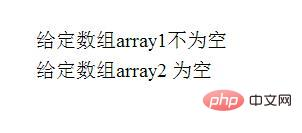# 如何使用PHP检查数组是否为空？（代码示例）empty()函数可以用来确定给定变量是否为空。如果变量不存在，则此函数不会返回警告。

`empty( \$var )`

```<?php
// 声明数组并初始化它
\$array1 = array('0' => 'hello','1' => 'php');

// 声明空数组
\$array2 = array();

// 检查数组的条件是否为空
if(!empty(\$array1))
echo "给定数组array1不为空 <br>";

if(empty(\$rray2))
echo "给定数组array2 为空";
?>```count()函数是用于计算数组中的所有元素。如果数组中的元素数为零，则它将显示空数组。

`count( \$array_or_countable )`

```<?php
// 声明一个空数组
\$empty_array = array();

// 检查数组是否为空
if(count(\$empty_array) == 0)
echo "数组为空";
else
echo "数组不为空";
?>```

`数组为空`

sizeof()函数用于检查数组的大小。如果数组的大小为零，则数组为空，否则数组不为空。

```<?php
// 声明一个空数组
\$empty_array = array();

if( sizeof(\$empty_array) == 0 )
echo "数组为空";
else
echo "数组不空";
?>```

`数组为空`